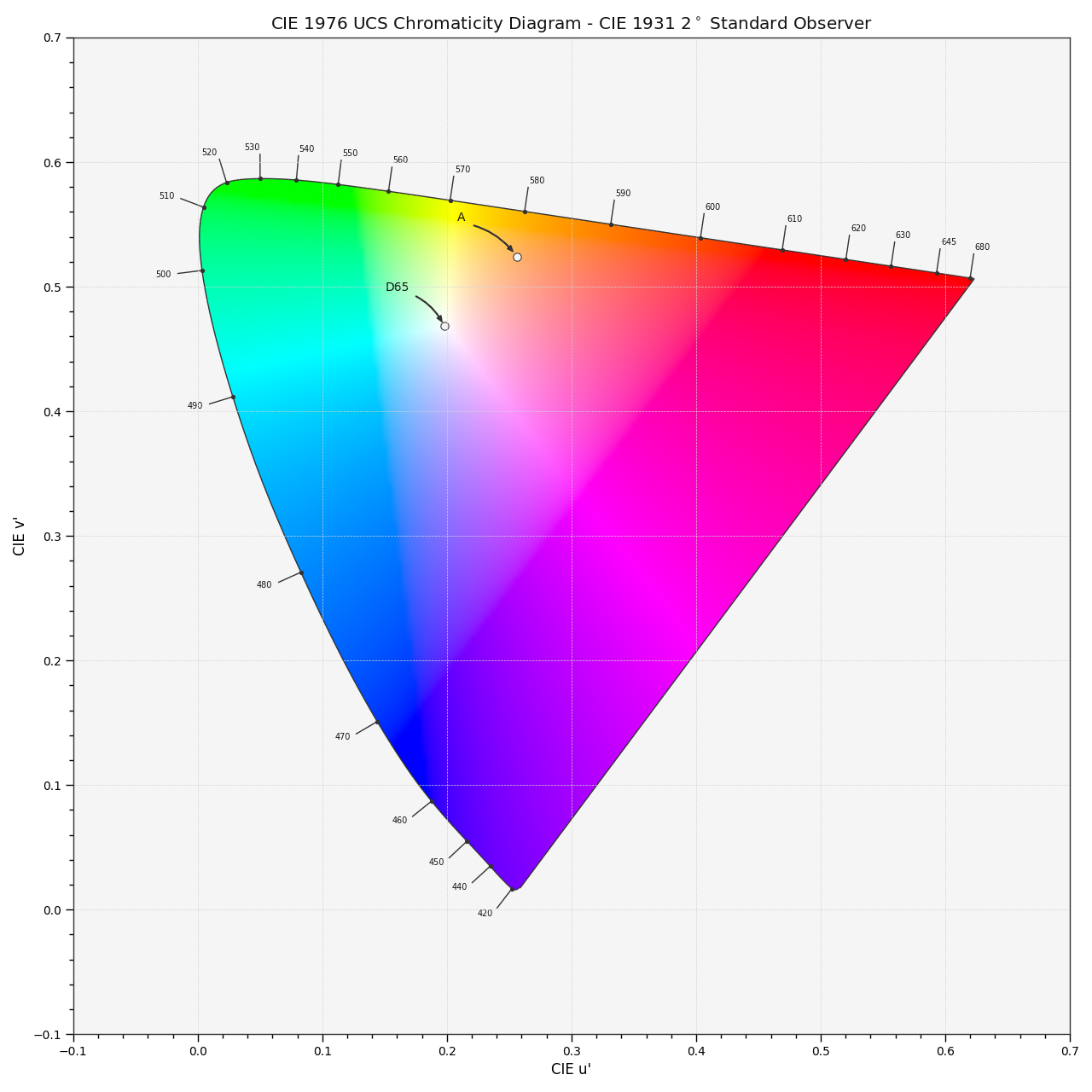# colour.plotting.plot_sds_in_chromaticity_diagram_CIE1976UCS#

colour.plotting.plot_sds_in_chromaticity_diagram_CIE1976UCS(sds: , cmfs: = 'CIE 1931 2 Degree Standard Observer', chromaticity_diagram_callable_CIE1976UCS: Callable = plot_chromaticity_diagram_CIE1976UCS, annotate_kwargs: Optional[Union[dict, List[dict]]] = None, plot_kwargs: Optional[Union[dict, List[dict]]] = None, **kwargs: Any) [source]#

Plot given spectral distribution chromaticity coordinates into the CIE 1976 UCS Chromaticity Diagram.

Parameters:
Returns:

Current figure and axes.

Return type:

`tuple`

Examples

```>>> A = SDS_ILLUMINANTS["A"]
>>> D65 = SDS_ILLUMINANTS["D65"]
>>> plot_sds_in_chromaticity_diagram_CIE1976UCS([A, D65])
...
(<Figure size ... with 1 Axes>, <...AxesSubplot...>)
```